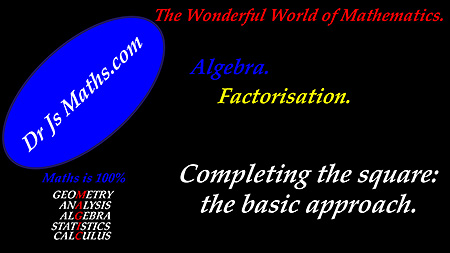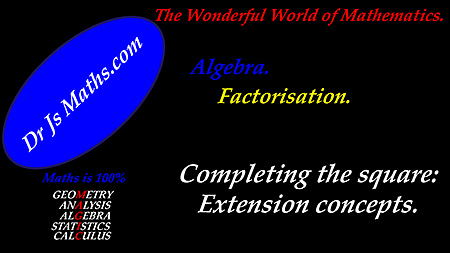Dr. J's Maths.com
Where the techniques of Maths
are explained in simple terms.

Algebra - Factorisation - Completing the Square.

The factorisation technique referred to as "Completing the Square" is one of the most versatile techniques both

• for factorising a quadratic expression; and

• for solving a quadratic equation.

It is certainly one of the aspects of mathematics you should be able to do really well. It will be referenced in many topics and in a diverse range of solutions.

Materials of direct relevance to the development of your understanding and your competence in the technique can be accessed by using the links below:

 Learning area. Resource Completing the square - the basic approach.Completing the square - extension of the basic technique to: odd coefficients for the x-term; non-monic quadratics.Summary of the steps to follow when implementing the method. Test Yourself. Complete the square - various types - Test Yourself 1. Complete the square - various types - Test Yourself 1 - Solutions. Complete the square - various types - Test Yourself 2. Complete the square - various types - Test Yourself 2 - Solutions.